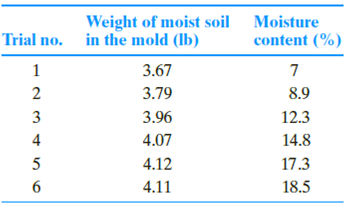Chapter 6, Problem 6.6PPrinciples of Geotechnical Enginee...

9th Edition
Braja M. Das + 1 other
ISBN: 9781305970939

Solutions

Chapter
SectionPrinciples of Geotechnical Enginee...

9th Edition
Braja M. Das + 1 other
ISBN: 9781305970939
Textbook Problem

Repeat Problem 6.4 with the following data (use Gs = 2.73):(a)

To determine

The maximum dry unit weight of compaction and the optimum moisture content.

Explanation

Given information:

The volume of mold (V) is 130ft3.

Calculation:

Calculate the unit weight of soil mass for trial 1 using the relation.

γ=WV

Here, W is the weight of moist soil in the mold for trial 1.

Substitute 3.67 lb for W and 130ft3 for V.

γ=3.67130=110.1lb/ft3

Similarly, calculate the unit weight of soil mass for remaining trials.

Calculate the dry unit weight of soil mass for trial 1 using the relation.

γd=γ1+w100

Here, w is the moisture content for trial 1.

Substitute 110.1lb/ft3 for γ and 7.0 % for w.

γd=110.11+7.0100=102.9lb/ft3

Similarly, calculate the dry unit weight of soil mass for remaining trials.

Summarize the calculated values of unit weight and dry unit weights as in Table (1)

(b)

To determine

The void ratio and degree saturation at the optimum moisture content.

Still sussing out bartleby?

Check out a sample textbook solution.

See a sample solution

The Solution to Your Study Problems

Bartleby provides explanations to thousands of textbook problems written by our experts, many with advanced degrees!

Get Started

What is a simple interest rate?

Engineering Fundamentals: An Introduction to Engineering (MindTap Course List)

How do you translate business rules into data model components?

Database Systems: Design, Implementation, & Management

Will online transactions eventually replace person-to-person contact? Why or why not?

Systems Analysis and Design (Shelly Cashman Series) (MindTap Course List)

Solve Problem 8.4-4 by the ultimate strength method (use the tables in Part 8 of the Manual).

Steel Design (Activate Learning with these NEW titles from Engineering!)

Voltage can be described as ____________ .

Welding: Principles and Applications (MindTap Course List)